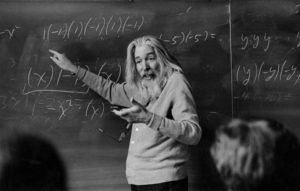# RIP Dr. Raymond Smullyan

Today I noticed an New York Times obituary for mathematician Raymond Smullyan. I was immediately drawn in by the photo of Smullyan demonstrating some very basic algebra.Smullyan was a college dropout and autodidact. I was amused to read an account of his academic history.

One of Smullyan’s teachers at the University of Chicago…Rudolf Carnap…recommended Smullyan for a mathematics post at Dartmouth College.. Smullyan had no formal qualifications at this time but was already working on mathematical research for future publications. He taught at Dartmouth College from 1954 until 1956, being awarded his B.S. from the University of Chicago in 1955. He had never completed sufficient courses to merit the award, but to make up the number Chicago credited him with a calculus course which he had never taken but was teaching.

The NY Times published a quiz of Smullyan’s math puzzles, but I was terribly disappointed at one question, presented as a logic puzzle. Here it is, with a correct solution reached by an incorrect procedure.That is utterly ridiculous. Any high school senior will immediately recognize this as a basic algebra question, not a logic question at all. It is hardly worthy of Smullyan’s legacy. Here is the proper method of solution, with nice graphics produced by Wolfram Alpha.

Edit: I decided the solution needs more explanation. We are told the price of a large bird (represented as x) equals the price of two small birds (2y). So we get (x = 2y). Then we found the price of five large birds (5x) and three small (3y) is (5x + 3y), the same as three large and five small, plus 20.  Since 3x + 5y is 20 less than 5x + 3y we add 20 to make them equal as (3x + 5y + 20). We could simplify that equation to (x = y + 10) but the Wolfram solver is just as happy to work with our original, unsimplified equations.

Result: {x = 2y, 5x + 3y = 3x + 5y + 20}

The two equations represented by lines will intersect at only one point, which is a solution that works for both equations.This is a simple solution of two simultaneous linear equations. It may look complex, but it’s basic Algebra 1. It is merely a literal translation of the problem into equations. It could be solved without plotting by substitution, but graphs are always fun (especially if I don’t have to plot them myself).

I was expecting a spark of genius, and ended up back in high school. But perhaps that is not so bad. I was recently employed as a mathematician, one of my colleagues gave me this XKCD cartoon. I understand it completely.